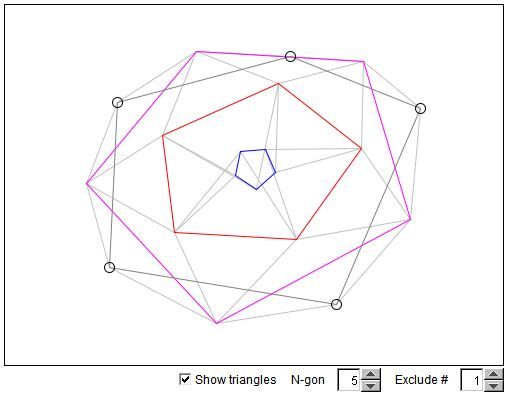# Douglas' Theorem

### If you are reading this, your browser is not set to run Java applets. Try IE11 or Safari and declare the site https://www.cut-the-knot.org as trusted in the Java setup.What if applet does not run?

I shall follow the articles by B.H.Neumann (1942) and J.Douglas (1940). (K. Petr published a proof of the result discussed below in 1905.) The theorem's most recent designation is PDN, Petr-Douglas-Newmann, giving credit to all three authors.)

Let the vertices of an n-gon be ordered A1, A2, ..., An and considered as complex numbers. We shall apply a certain operation to every pair of consecutive vertices.

For any two complex numbers A and B, the all-important operation is

 (1) C = (1 - c)A + cB

If c is real, points C lie on a straight line through A and B. For complex c, A,B, and C form a triangle similar to the triangle formed by points 0, 1, and c. The orientation is preserved. If c = λ + iμ, then to construct C, draw a line through (1 - λ)A + λB perpendicular to AB and mark a point at the distance μ|B - A| from AB.

When applied to all pairs of successive vertices of an n-gon P, operation (1) yields another polygon, Pc. In geometric terms, Pc consists of the apexes of similar triangles erected on the sides of the polygon P. Vertices of Pc are generated successively from those of P. Jesse Douglas calls (1) a linear polygonal transformation - LPT. LPT has several important properties. First, P and Pc are concentric, i.e. share the centroid.

 (2) ∑Pi = ∑(Pc)i

To obtain other properties, arrange vertices of P and Pc as n-dimensional vectors. (1) gets then represented as a linear transformation with an n×n circulant matrix Lc with the first row given by {(1 - c) c 0 ...0}. A general circulant matrix M can also be defined by its first row { α0  α1 ... αn-1}. Let K denoted the simplest circulant {0 1 0 ... 0}. Then Kn = I (a unit matrix) and

M = α0I + α1K + α2K2 + ... + αn-1Kn-1

or M = pM(K). pM(t) is known as the auxiliary polynomial of M. When two circulants are multiplied, their auxiliary polynomials are multiplied modulo Kn - I. This shows that any two circulant matrices commute, and so are LPTs. From here we also obtain a fact that is not quite obvious from geometric considerations, viz., the result of a sequence of LPTs does not depend on the order of individual transformations.

The auxiliary polynomial of a product of several LPTs is simply a product of the terms ((1 - c) + ct). For the generalization of Napoleon's theorem we are going to select LPTs in a particular manner.

Let ωd denote (n - 1) distinct roots of unity ωn = 1, excluding 1. In other words, ωd's are all roots of the equation

 (3) r(t) = tn-1 + tn-2 + ... + t + 1 = 0

For d = 1, 2, ..., n-1, define

 (4) cd = 1/(1 - ωd)

Verify that cd = (1 + i·cot(πd/n))/2 and also (1 - cd) + tcd = (t - ωd)/(1 - ωd).

With thus selected cd's, (1) is equivalent to a construction of isosceles triangles with simple apex angles. (Note that for n = 3, the angles are ±120°). From (3) and (4) we have

((1 - c1) + c1t)...((1 - cn-1) + cn-1t) = r(t)/r(1) = r(t)/n

which corresponds to the circulant matrix {1/n 1/n 1/n... 1/n}. This matrix transforms any vector-polygon A1...An into the centroid of points A1, ..., An.

Omit now any of the constituent LPTs. Let it be (1) with cd for some d. (n - 2) LPTs remain. Since LPTs commute, the polygon obtained after (n - 2) steps has the property that a single application of (1) with cd shrinks it to a point. Which exactly means that the polygon is either regular or star-shaped.

For n = 3, we get the inner or outer Napoleon triangles depending on whether c1 or c2 is omitted.

Context